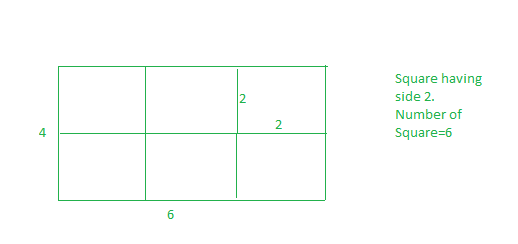# Minimum squares to evenly cut a rectangle

Given a rectangular sheet of length l and width w. we need to divide this sheet in the square sheets such that the number of square sheets should be as minimum as possible.

Examples:

Input :l= 4 w=6
Output :6
We can form squares with side of 1 unit, But the number of squares will be 24, this is not minimum. If we make square with side of 2, then we have 6 squares. and this is our required answer.
And also we can’t make square with side 3, if we select 3 as square side, then whole sheet can’t be converted into suares of equal length.Input :l=3 w=5
Output :15

## Recommended: Please try your approach on {IDE} first, before moving on to the solution.

Optimal length of side of a square is equal to GCD of two numbers

## C++

 `// CPP program to find minimum number of ` `// squares to make a given rectangle. ` `#include ` `using` `namespace` `std; ` ` `  `int` `countRectangles(``int` `l, ``int` `w) ` `{ ` `    ``// if we take gcd(l, w), this ` `    ``// will be largest possible ` `    ``// side for suare, hence minimum ` `    ``// number of square. ` `    ``int` `squareSide = __gcd(l, w); ` ` `  `    ``// Number of squares. ` `    ``return` `(l * w) / (squareSide * squareSide); ` `} ` ` `  `// Driver code ` `int` `main() ` `{ ` `    ``int` `l = 4, w = 6; ` `    ``cout << countRectangles(l, w) << endl; ` `    ``return` `0; ` `} `

## Java

 `// Java program to find minimum number of ` `// squares to make a given rectangle. ` ` `  `class` `GFG{ ` `static` `int` `__gcd(``int` `a, ``int` `b) { ` `   ``if` `(b==``0``) ``return` `a; ` `   ``return` `__gcd(b,a%b); ` `} ` `static` `int` `countRectangles(``int` `l, ``int` `w) ` `{ ` `    ``// if we take gcd(l, w), this ` `    ``// will be largest possible ` `    ``// side for suare, hence minimum ` `    ``// number of square. ` `    ``int` `squareSide = __gcd(l, w); ` ` `  `    ``// Number of squares. ` `    ``return` `(l * w) / (squareSide * squareSide); ` `} ` ` `  `// Driver code ` `public` `static` `void` `main(String[] args) ` `{ ` `    ``int` `l = ``4``, w = ``6``; ` `    ``System.out.println(countRectangles(l, w)); ` `} ` `} ` `// This code is contributed by mits `

## Python3

 `# Python3 code to find minimum number of ` `# squares to make a given rectangle. ` ` `  `import` `math  ` ` `  `def` `countRectangles(l, w): ` ` `  `    ``# if we take gcd(l, w), this ` `    ``# will be largest possible ` `    ``# side for suare, hence minimum ` `    ``# number of square. ` `    ``squareSide ``=` `math.gcd(l,w) ` `     `  `    ``# Number of squares. ` `    ``return` `(l``*``w)``/``(squareSide``*``squareSide) ` ` `  `# Driver Code ` `         `  `if` `__name__ ``=``=` `'__main__'``: ` `    ``l ``=` `4` `    ``w ``=` `6` `    ``ans ``=` `countRectangles(l, w) ` `    ``print` `(``int``(ans)) ` ` `  `# this code is contributed by ` `# SURENDRA_GANGWAR `

## C#

 `// C# program to find minimum number of ` `// squares to make a given rectangle. ` ` `  `class` `GFG{ ` `static` `int` `__gcd(``int` `a, ``int` `b) { ` `if` `(b==0) ``return` `a; ` `return` `__gcd(b,a%b); ` `} ` `static` `int` `countRectangles(``int` `l, ``int` `w) ` `{ ` `    ``// if we take gcd(l, w), this ` `    ``// will be largest possible ` `    ``// side for suare, hence minimum ` `    ``// number of square. ` `    ``int` `squareSide = __gcd(l, w); ` ` `  `    ``// Number of squares. ` `    ``return` `(l * w) / (squareSide * squareSide); ` `} ` ` `  `// Driver code ` `public` `static` `void` `Main() ` `{ ` `    ``int` `l = 4, w = 6; ` `    ``System.Console.WriteLine(countRectangles(l, w)); ` `} ` `} ` `// This code is contributed by mits `

## PHP

 ` `

Output:

```6
```

Attention reader! Don’t stop learning now. Get hold of all the important DSA concepts with the DSA Self Paced Course at a student-friendly price and become industry ready.

My Personal Notes arrow_drop_upCheck out this Author's contributed articles.

If you like GeeksforGeeks and would like to contribute, you can also write an article using contribute.geeksforgeeks.org or mail your article to contribute@geeksforgeeks.org. See your article appearing on the GeeksforGeeks main page and help other Geeks.

Please Improve this article if you find anything incorrect by clicking on the "Improve Article" button below.

Article Tags :
Practice Tags :

Be the First to upvote.

Please write to us at contribute@geeksforgeeks.org to report any issue with the above content.